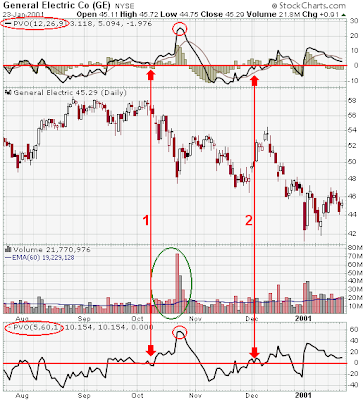# Percentage Volume Oscillator (PVO)

Introduction and Calculation
The Percentage Volume Oscillator (PVO) is the percentage difference between two moving averages of volume. The indicator is calculated with the following formula:
PVO = ((Shorter EMA of Volume - Longer EMA of Volume) / Longer EMA of Volume) x 100
The PVO oscillates around zero.

## Uses

The PVO can be used to identify periods of expanding or contracting volume in three different ways:
• Centerline Crossovers: like the PPO, the PVO oscillates above and below the zero line. When PVO is positive, the shorter EMA of volume is greater than the longer EMA of volume. When PVO is negative, the shorter EMA of volume is less than the longer EMA of volume. A PVO above zero indicates that volume levels are generally above average and relatively heavy. When the PVO is below zero, volume levels are generally below average and light.
• Directional Movement: General directional movement of the PVO can offer a quick visual assessment of volume patterns. A rising PVO signals that volume levels are increasing and a falling PVO signals that volume levels are decreasing.
• Moving Average Crossovers: The last variable in the PVO forms the signal line. For example: PVO(12,26,9) would include a 9-day EMA of PVO as well as a histogram representing the difference between the PVO and its 9-day EMA. When PVO moves above its signal line, volume levels are generally increasing. When PVO moves below its signal line, volume levels are generally decreasing.
Movements in the PVO are completely separate from price movements. As such, movements in PVO can correlated with price movements to assess the degree of buying or selling pressure. Advances combined with strength in the PVO would be considered strong. Should the PVO decline while a security's price fell, it would indicate decreasing volume on the decline.

## ExamplesIn the example above, FILE is shown with two PVO settings: PVO (12,26,9) in the top window and PVO (5,60,1) in the bottom window. When the final variable is set at 1, as with PVO(5,60,1) there is no signal line or histogram. During August and September, the stock traded between 15 and 21, and the PVO remained mostly below zero. There was a small bounce above zero with the late August advance, but the stock remained confined to its trading range. When the stock began to advance off of its low in October, the PVO moved into positive territory with a sharp rise (green line). The advance was confirmed with expanding volume and the stock broke resistance. The breakout with expanding volume signaled exceptionally strong buying pressure.In the example above, the PVO is set in the top window at the default setting (12,26,9) and in the bottom window at (5,60,1). Even though the line shapes for both PVO settings are almost identical, the scales on the right reflect different ranges and crossover points.
• PVO (12,26,9) surpassed +20 in late October, while PVO (5,60,1) surpassed +50.
• In early October (red line #1), PVO (5,60,1) crossed below zero, but PVO (12,26,9) remained above.
• At the beginning of December (red line #2), PVO (5,60,1) moved above zero before PVO (12,26,9) did.
Much of this difference can be attributed to the short EMA of volume in both PVO settings. The 5-day EMA of volume is much more sensitive than the 12-day EMA of volume. Shorter moving averages are more volatile and more likely to have centerline crossovers. Above-average volume periods can also be confirmed by watching for volume bars that exceed the 60-day EMA (green oval in October). Notice that both PVO's shot up in the second half of October as volume spiked above 60m shares.

## PVO and SharpChartsUsing SharpCharts, PVO can be set as an indicator above or below a security's price plot. Once the indicator is chosen from the drop down list, the three boxes to the right are used to adjust the settings. The first box is for the short exponential moving average (EMA) of volume, the second is for the long exponential moving average of volume and the third is for the signal line. The default setting (12,26,9) uses a 12-day EMA of volume and a 26-day EMA of volume to calculate the PVO and a 9-day EMA of PVO as the signal/trigger line. For those who do not wish to have a trigger line or histogram, the third box can be set equal to 1. PVO appears as the thick solid line and the signal/trigger line as the thinner and smoother line.